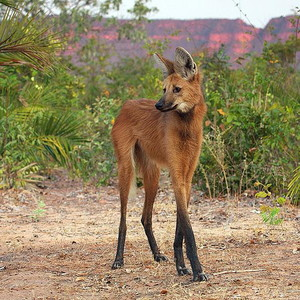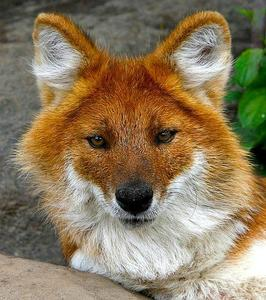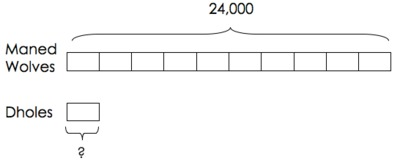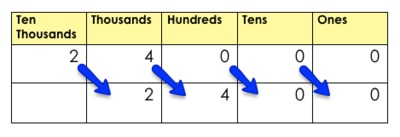# Threatened and Endangered

Alignments to Content Standards: 4.NBT.A.1 4.OA.A.1

Maned wolves are a threatened species that live in South America. People estimate that there are about 24,000 of them living in the wild.The dhole is an endangered species that lives in Asia. People estimate there are ten times as many maned wolves as dholes living in the wild.About how many dholes are there living in the wild?

## IM Commentary

This task addresses 4.NBT.1 in that in requires students to understand multiplying and dividing whole numbers by powers of 10. It also addresses 4.OA.1 since it helps students push their understanding of multiplication from a 3rd grade understanding of repeated addition to a 4th grade understanding that we can also use multiplication to understand "times as many" comparison problems.

A tape-diagram may be helpful in this problem. Though this task seems straightforward, it actually requires students to work backwards from the known value that is 'ten times as many.' Students may impulsively want to multiply the number of maned wolves by a factor of 10. The tape diagram will help students organize the different numbers and animals in this problem. A challenging follow-up task to this one is 4.NBT Millions and Billions of People.

Population facts from http://wwf.panda.org/

## Solutions

Solution: 1

There are about 24,000=20,000+4,000 maned wolves. 20,000 is ten times as many as 2,000, and 4,000 is ten times as many as 400. So there are about 2,000+400 or 2,400 dholes estimated to live in the wild.

We can check this:

$$10\times(2000+400)=10\times2,000+10\times400=20,000+4,000=24,000$$

Solution: 2

We can also use a tape diagram to show that the number of maned wolves (24,000) is ten times the number of dholes. The number of dholes must be 2,400 (which a student could then check with multiplication or repeated addition.)For a student to use this strategy effectively, s/he would have to have some understanding of how to divide 24,000 by 10.

For students struggling to figure out the division, it may help to model the following thought process: "Could one unit be worth 1,000 animals...2,000 animals...3,000 animals?" Understanding that each unit must represent somewhere between 2,000 and 3,000 animals would be a likely first step.

From there, the thought process might be: "If we know each unit represents 2,000 animals, then we still need to account for 4,000 more animals. Could one unit contain an additional 100 animals...200 animals...300 animals...400 animals...500 animals?"

Through this reasoning, students could come to the conclusion that each unit must represent 2,400 animals.

Solution: 3

We can also use a place value organizer to think about dividing 24,000 by 10.We know that no matter the number in our base ten system, if we divide by 10 then every digit will shift to the right in place value. This place value organizer shows how we can represent dividing 24,000 by 10 using place value.Using this organizer, we can see that 24,000 divided by 10 is 2,400. Therefore, there are about 2,400 dholes.